### Hyperelastic Materials: Principal Stresses of Isotropic Hyperelastic Materials

Careful investigation of the Cauchy stress matrix (See Equation 2, Equation 3, and Equation 4) of the three forms of strain energy functions of Isotropic Hyperelastic materials studied above shows the following: For the first and third form, when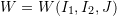or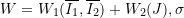admits the form: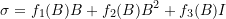where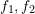, and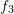are some scalar valued functions of the invariants of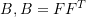is the Left Cauchy-Green deformation tensor. Similarly, for the second form, when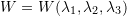, thenadmits the form: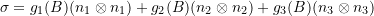where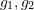, and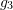are scalar valued functions of the invariants of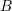. Note that the to obtain the above forms, the fact that the invariants of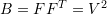and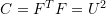are equal was utilized and that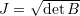. As shown earlier, the three forms of the strain energy functions are equivalent and therefore, the expressions for the Cauchy stress matrix are also equivalent. Any of these two expressions imply that the eigenvectors of \sigma are aligned with the eigenvectors of B which are also aligned with the eigenvectors of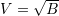. Note that to reach this conclusion, we utilized the fact that the eigenvectors of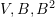and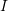are all aligned since: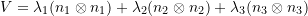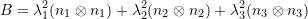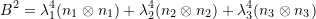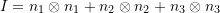The intuitive explanation of this observation is that for a deformation gradient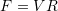, the material vectors are first rotated by the tensor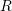. Then, they are stretched by the positive definite symmetric matrix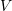. Because of isotropy, the resulting principal stresses are aligned with the eigenvectors ofas well. In other words, the principal directions of stretch are those directions that have normal stresses and zero shear stresses.